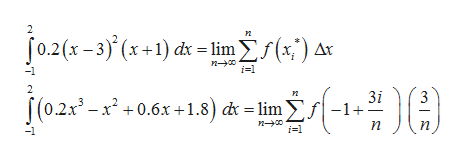# Use:F(x)= 0.2((x-3)^2)(x+1), bounded by the x axis, over [-1,2]Use the limit definition of Riemman Sum to find the earea shaded beneath the curve, round to 3 decimal places.

Question
23 views

Use:

F(x)= 0.2((x-3)^2)(x+1), bounded by the x axis, over [-1,2]

Use the limit definition of Riemman Sum to find the earea shaded beneath the curve, round to 3 decimal places.

check_circle

Step 1

The given function is,

Step 2

The length of the sub-intervals and the function is computed as follows,

Step 3

The formula for computing the a...help_outlineImage Transcriptionclose[0.2(x - 3)° (x +1) đx = lim f(x;') ax i=1 3i |(0. S(0.2x -x* + 0.6x +1.8) d = lim S -1+- C i=1 -1 fullscreen

### Want to see the full answer?

See Solution

#### Want to see this answer and more?

Solutions are written by subject experts who are available 24/7. Questions are typically answered within 1 hour.*

See Solution
*Response times may vary by subject and question.
Tagged in

### Functions